Courses

# Solutions of Magnetic Effects of Electric Current (Page No- 73) - Physics By Lakhmir Singh, Class 10 Class 10 Notes | EduRev

## Class 10 : Solutions of Magnetic Effects of Electric Current (Page No- 73) - Physics By Lakhmir Singh, Class 10 Class 10 Notes | EduRev

The document Solutions of Magnetic Effects of Electric Current (Page No- 73) - Physics By Lakhmir Singh, Class 10 Class 10 Notes | EduRev is a part of the Class 10 Course Lakhmir Singh & Manjit Kaur Solutions: Class 10 Science.
All you need of Class 10 at this link: Class 10

Page No:73

Question 1: State any two properties of magnetic field lines.

Solution : Prop of magnetic field lines:
(i) The magnetic field lines originate from the north pole of a magnet and end at its south pole.
(ii) The strength of magnetic field is indicated by the degree of closeness of the field lines. Where the field lines are closest together, the magnetic field is the strongest there.

Question 2: What are the two ways in which you can trace the magnetic field pattern of a bar magnet ?

Solution : (i) By using iron filings
(ii) By using compass

Question 3: You are given the magnetic field pattern of a magnet. How will you find out from it where the magnetic field is the strongest ?

Solution : Magnetic field is the strongest at the place where the magnetic field lines are closest together.

Question 4: State whether the following statement is true or false :
The axis of earth’s imaginary magnet and the geographical axis coincide with each other.

Solution :
False
The axis of earth’s imaginary magnet is inclined at an angle of 15o with the geographical axis.

Question 5: Why does a compass needle get deflected when brought near a bar magnet ?

Solution : A compass needle gets deflected when brought near a bar magnet because the bar magnet exerts a magnetic force on the compass needle, which is itself a tiny pivoted magnet.

Question 6: Where do the manufacturers use a magnetic strip in the refrigerator ? Why is this magnetic strip used ?

Solution : Manufacturers use a magnetic strip in the refrigerator’s door to keep it closed properly.

Question 7:
Fill in the following blanks with suitable words :
(a) Magnetic field lines leave the………….. pole of a bar magnet and enter at its……………
(b) The earth’s magnetic field is rather like that of a………….. magnet with its……….. pole in the northern hemisphere.

Solution :
(a) north; south.
(b) bar; south.

Question 8: Draw a diagram to show the magnetic field lines around a bar magnet.

Solution :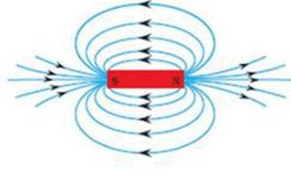Magnetic field lines around a bar magnet.

Question 9: What is a magnetic field ? How can the direction of magnetic field lines at a place be determined ?

Solution : The space surrounding a magnet in which magnetic force is exerted, is called a magnetic field. The direction of magnetic field lines at a place can be determined by using a compass needle. A compass needle placed near a magnet gets deflected due to the magnetic force exerted by the magnet. The north end of the needle of the compass indicates the direction of magnetic field at the point where it is placed.

Question 10: Explain why, two magnetic field lines do not intersect each other.

Solution : Two magnetic field lines do not intersect each other due to the fact that the resultant force on a north pole at any point can be only in one direction. But if the two magnetic lines get intersect one another, this means that resultant force on a north pole placed at the point of interection will be along two directions, which is not possible.

Question 11: When an electric current is passed through any wire, a magnetic field is produced around it. Then why an electric iron connecting cable does not attract nearby iron objects when electric current is switched on through it ?

Solution : Because the strength of magnetic field produced by the cable is quite weak.

Question 12: (a) Define magnetic field lines. Describe an activity to draw a magnetic field line outside a bar magnet from one pole to another pole.
(b) Explain why, a freely suspended magnet always points in the north-south direction.

Solution : (a) The magnetic field lines are the lines drawn in a magnetic field along which a north magnetic pole would move. The magnetic field lines are also known as magnetic lines of forces.
Activity to draw a magnetic field line outside a bar magnet from one pole to another pole:
Take a small compass and a bar magnet.
Place the magnet on a sheet of white paper fixed on a drawing board, using some adhesive material.
Mark the boundary of the magnet.
Place the compass near the north pole of the magnet. The south pole of the needle points towards the north pole of the magnet. The north pole of the compass is directed away from the north pole of the magnet.
Mark the position of two ends of the needle.
Now move the needle to a new position such that its south pole occupies the position previously occupied by its north pole.
In this way, proceed step by step till you reach the south pole of the magnet .
Join the points marked on the paper by a smooth curve. This curve represents a magnetic field line.
(b) A freely suspended magnet points in the north-south direction because earth behaves as a magnet with its south pole in the geographical north and the north pole in the geographical south.

Page No:74

Question 24: Copy the figure given below which shows a plotting compass and a magnet. Label the N pole of the magnet and draw the field line on which the compass lies.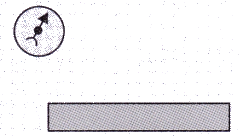Solution : As the north pole of the magnetic needle is pointing in the opposite direction,so the nearer end of the magnet will be north pole.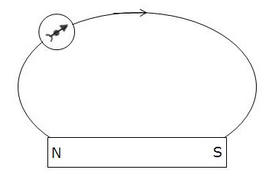Question 25: (a) The diagram shows a bar magnet surrounded by four plotting compasses. Copy the diagram and mark in it the direction of the compass needle for each of the cases B, C and D.
(b) Which is the north pole, X or Y ?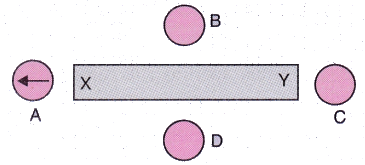Solution :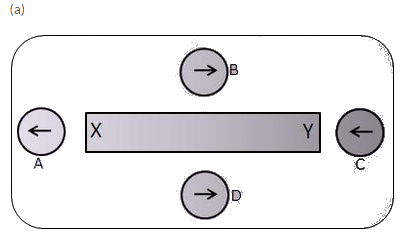(b) X, as it repels the north pole (tip) of magnetic needle.

Question 26: The three diagrams in the following figure show the lines of force (field lines) between the poles of two magnets. Identify the poles A, B, C, D, E and F.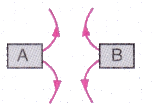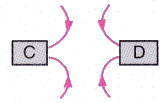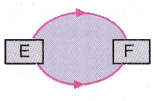Solution : A = N; B = N; C = S; D = S; E = N; F = S

Question 27: The figure given below shows the magnetic field between two magnets :
(i) Copy the diagram and label the other poles of the magnets.
(ii) Which is the weaker magnet ?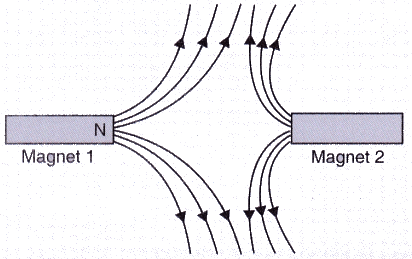Solution :
(a)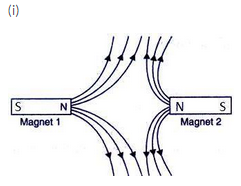(ii) Magnet 2 is weaker.

Offer running on EduRev: Apply code STAYHOME200 to get INR 200 off on our premium plan EduRev Infinity!

61 docs

,

,

,

,

,

,

,

,

,

,

,

,

,

,

,

,

,

,

,

,

,

,

,

,

;